Next: Conditional probability and Bayes' Up: Probability Previous: Rules of probability   Contents

## Subjective probability and objective'' description of the physical world

The subjective definition of probability seems to contradict the aim of physicists to describe the laws of physics in the most objective way (whatever this means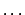). This is one of the reasons why many regard the subjective definition of probability with suspicion (but probably the main reason is because we have been taught at university that probability is frequency''). The main philosophical difference between this concept of probability and an objective definition that we would have liked'' (but which does not exist in reality) is that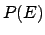is not an intrinsic characteristic of the event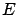, but depends on the state of information available to whoever evaluates. The ideal concept of objective'' probability is recovered when everybody has the same'' state of information. But even in this case it would be better to speak of intersubjective probability. The best way to convince ourselves about this aspect of probability is to try to ask practical questions and to evaluate the probability in specific cases, instead of seeking refuge in abstract questions. I find, in fact, that -- to paraphrase a famous statement about Time -- Probability is objective as long as I am not asked to evaluate it.'' Here are some examples.
Example 1:
What is the probability that a molecule of nitrogen at room temperature has a velocity between 400 and 500 m/s?''. The answer appears easy: take the Maxwell distribution formula from a textbook, calculate an integral and get a number''. Now let us change the question: I give You a vessel containing nitrogen and a detector capable of measuring the speed of a single molecule and You set up the apparatus (or You let a person You trust do it). Now, what is the probability that the first molecule that hits the detector has a velocity between 400 and 500 m/s?''. Anybody who has minimal experience (direct or indirect) of experiments would hesitate before answering. He would study the problem carefully and perform preliminary measurements and checks. Finally he would probably give not just a single number, but a range of possible numbers compatible with the formulation of the problem. Then he starts the experiment and eventually, after 10 measurements, he may form a different opinion about the outcome of the eleventh measurement.
Example 2:
What is the probability that the gravitational constant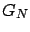has a value between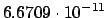and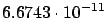m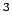kg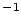s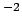?''. Before 1994 you could have looked at the latest issue of the Particle Data Book and answered that the probability was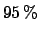. Since then -- as you probably know -- three new measurements ofhave been performed and we now have four numbers which do not agree with each other (see Tab.). The probability of the true value ofbeing in that range is currently dramatically decreased.

Table: Results of measurements of the gravitational constant.
 Institute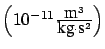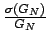(ppm)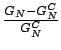(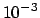) CODATA 1986 (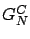'')128 - PTB (Germany) 1994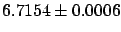83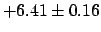MSL (New Zealand) 1994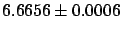95Uni-Wuppertal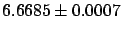105(Germany) 1995

Example 3:
What is the probability that the mass of the top quark, or that of any of the supersymmetric particles, is below 20 or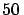GeV?''. Currently it looks as if it must be zero. Ten years ago many experiments were intensively looking for these particles in those energy ranges. Because so many people where searching for them, with enormous human and capital investment, it meant that, at that time, the probability was considered rather high: high enough for fake signals to be reported as strong evidence for them3.4.
The above examples show how the evaluation of probability is conditioned by some a priori (theoretical'') prejudices and by some facts (experimental data''). Absolute'' probability makes no sense. Even the classical example of probability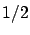for each of the results in tossing a coin is only acceptable if: the coin is regular, it does not remain vertical (not impossible when playing on the beach), it does not fall into a manhole, etc.

The subjective point of view is expressed in a provocative way by de Finetti's

PROBABILITY DOES NOT EXIST''.Next: Conditional probability and Bayes' Up: Probability Previous: Rules of probability   Contents
Giulio D'Agostini 2003-05-15